# Geocaching Puzzle Caches: How to find the Orthocenter of a Triangle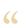How do I find the orthocenter of the triangle created by three waypoints?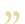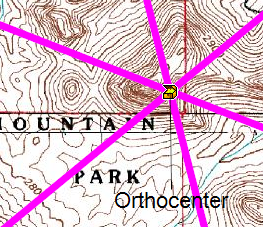The orthocenter of a triangle is located at the intersection of the three lines. Each line runs through a vertex and is perpendicular to the opposite side.

Let’s solve a geocaching puzzle cache that requires us to find the orthocenter of a triangle. By adding the three waypoints to a closed route in ExpertGPS or GeoBuddy, we can calculate the bearings along each edge of the triangle. By adding 90 degrees to each bearing, we can determine the direction of a line perpendicular to that edge. Now we can use the Project Waypoint dialog to project a line from the opposite vertex.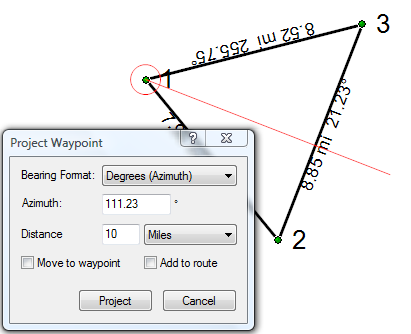In the second screenshot, I’m projecting a line from waypoint 1 at 111.23 degrees (which is 90 degrees more than the bearing of the opposite edge – 21.23 degrees). Repeat three times, creating a new route between the vertex and the newly-projected waypoint, and you’ve found the orthocenter.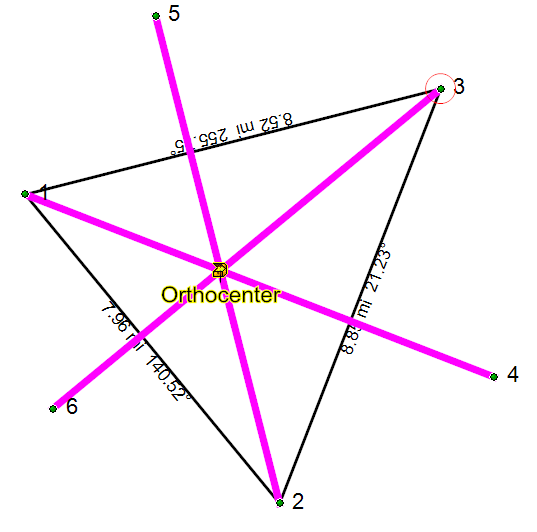### One Response to Geocaching Puzzle Caches: How to find the Orthocenter of a Triangle

1. Anthony says:

I’d like to set this up in my area, but to implement it, doesn’t it have to be worked in reverse? In other words, hide the cache, get the coordinates, and then find a way to make 3 waypoints forming a triangle, with the orthocenter being the coordinates of the cache?

To do so in reverse of that would be more difficult I would think, getting 3 waypoints, and then projecting lines to get the orthocenter, and then hide the cache at the center. But what if the orthocenter is not a suitable location for the cache? It would be near impossible to manipulate the triangle so that the orthocenter would be the coordinates you want.

So I guess the main question here is, can this be done in reverse? Generate 3 waypoints based on one set of coordinates in the middle?